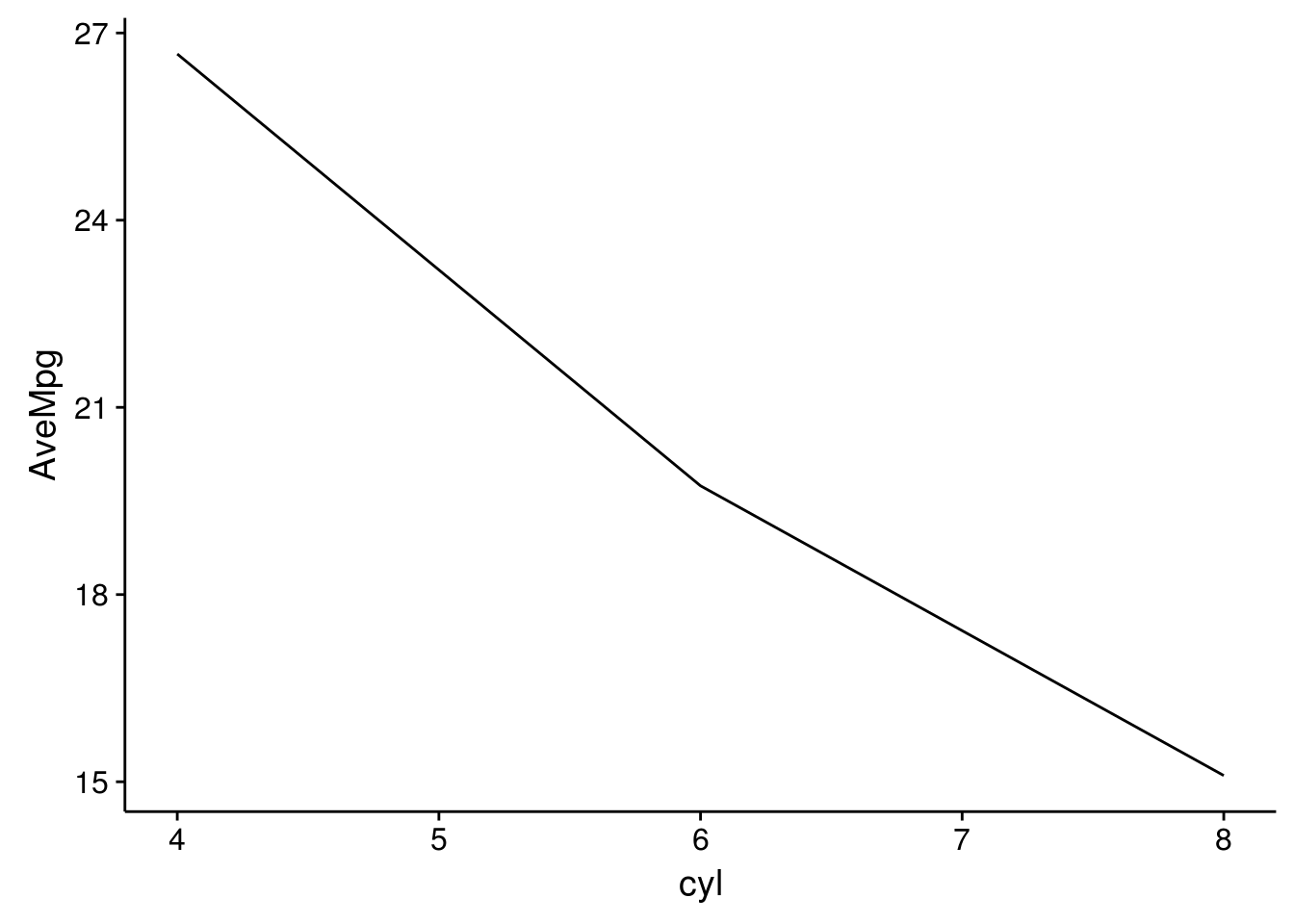Test-Blogdown
July 29, 2017
[ R ]
R blogdown

#### This is a test R blogdown post

``print("Hello World")``
``##  "Hello World"``
``##  "Hello World without code shown"``
``````test <- mtcars %>% group_by(cyl) %>%
summarize(AveMpg = mean(mpg))

ggplot(data=test, mapping=aes(x=cyl, y=AveMpg)) +
geom_line(stat="identity")``````• 2017/08/24 A Great Blogdown Tutorial
• 2016/12/30 A Plain Markdown Post